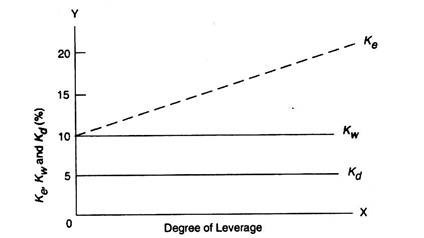# Net Operating Income Approach (NOI)

According to Net Operating Income Approach which is just opposite to NI approach, the overall cost of capital and value of firm are independent of capital structure decision and change in degree of financial leverage does not bring about any change in value of firm and cost of capital.

The Net approach suggest that the market value of the firm is not affected by the capital structure changes and overall cost of capital remains constant like NI approach, it is also based upon certain assumptions which are :

• The market capitalizes the value of the firms as a whole. Thus, the split between debt and equity is not important.
• The debt capitalization race (K0) remains constant and there is no corporate tax.

Ke = Ko + (Ko + Ki) D/E

Where D/E is the debt-equity ratio at market price. The equation 2 states that if Ko and ki are constant, then Ke will rise linearly with leverage. Thus there is no single point or range where the capital structure is optimum. Graphically, it can be represented as follows:Under the NOI approach when low cost debt is introduced in the capital structure, its advantages are exactly offset by the increased cost of equity in such a way that the resultant cost of capital remains same. Hence, there is no optimum capital remains same. Hence, there is no optimum capital structure or cost of capital is not a function of leverage.

### Features of NOI approach

1. At all degrees of leverage (debt), the overall capitalization rate would remain constant. For a given level of Earnings before Interest and Taxes (EBIT), the value of a firm would be equal to EBIT/overall capitalization rate.
2. The value of equity of a firm can be determined by subtracting the value of debt from the total value of the firm. This can be denoted as follows:

Value of Equity = Total value of the firm – Value of debt

1. Cost of equity increases with every increase in debt and the weighted average cost of capital (WACC) remain constant. When the debt content in the capital structure increases, it increases the risk of the firm as well as its shareholders. To compensate for the higher risk involved in investing in highly levered company, equity holders naturally expect higher returns which in turn increases the cost of equity capital.

### Assumptions of the Net Operating Income Approach (NOI)

(1) The firm is evaluated as a whole by the market. Accordingly, overall capitalization rate is used to calculate the value of the firm. The split of capitalization between debt and equity is not significant.

(2) Overall capitalization rate remains constant regardless of any change in degree of financial leverage.

(3) Use of debt as cheaper source of funds would increase the financial risk to shareholders who demand higher cost on their funds to compensate for the additional risk. Thus, the benefits of lower cost of debt are offset by the higher cost of equity.

(4) The cost of debt would stay constant.

(5) The firm does not pay income taxes.

## 2 thoughts on “Net Operating Income Approach (NOI)”

error: Content is protected !!